# finding Vbe in NPN Biasing

#### yef smith

Joined Aug 2, 2020
498

#### Irving

Joined Jan 30, 2016
3,444
The base-emitter junction isnt a resistor, its non-linear. All you can use, as a first approximation, is that there is roughly a 0.6v drop from b to e.

So R2 = (10 - 0.6)/Ib, where Ib is your desired base current.

#### BobTPH

Joined Jun 5, 2013
6,543
That is a terrible biasing scheme. The collector current can vary by as much as 3:1 depending on the actual beta of the transistor, which is not well controlled.

#### Papabravo

Joined Feb 24, 2006
20,162
Google "Voltage Divider Bias" for a more stable less sensitive biasing scheme. Use this method until you have a good reason not to do that.

#### MrChips

Joined Oct 2, 2009
28,551
Simulators do not give you the real life picture.
The base-emitter current shown is 6A. The transistor was dead a long time ago!

•nsaspook

#### crutschow

Joined Mar 14, 2008
32,088
But Vb is not always 0.7 i can change R2 to 0.01 in my schematics and get Vbe=9.9 V as shown bellow.
Of course, since you applying nearly a dead short from the supply to the base,
The base-emitter junction looks like a diode, so it will carry a very large current if there is not a significant resistance to limit it.
what is the strategy to calculate exactly the Vb number given by simulator?
You assume it is about 0.7V and then calculate the resulting collector and base currents.
From that you can look at the transistor data sheet for Vbe variation with base current to see if you need to tweak the voltage.
In most cases the difference between the assumed value and the actual value (likely no more than a tenth of a volt) is not enough to significantly change the bias values.

Last edited:

#### Audioguru again

Joined Oct 21, 2019
5,829
Your schematic in post #6 has the base of the transistor shorted to +9.9V.
Since the emitter is connected to ground then you have the Vbe diode with 9.9V across it.
Then all the current from the power supply will quickly burn out the base-emitter diode.

Your schematic in post #8 has way too much base current for the collector current to be 50mA with a collector voltage of 5V so that it can swing up and down with the signal at the base.
The collector voltage is shown to be only 0.257V.

Even if you selected resistors that allow a simulation to work properly, the circuit you make probably will not work because the transistor you use will have a different current gain, a different Vbe and a different temperature than the transistor in the simulation.
The circuit needs an emitter resistor or collector to base negative feedback resistor to reduce those differences.

#### yef smith

Joined Aug 2, 2020
498
Hello AudioGuru,at first i want to see the biasing theory of NPN to see the different states of the NPN
cutoff ,active,saturation.
NP is forward Biased when V_N<V_P because we push the majority carriers of electrons to the P side.
for cuttoff V_E>V_B and and V_C>V_B as shown bellow.

in saturation we got both our NP junction forawrd biased as shown bellow.
In active Emitter base junction is forward biased while the Base collector junction is backward biased
V_B < V_C and V_E<V_B (simulation shown bellow)

the problem is i dont see any difference between the saturation and active states.
They both have almost the same current in the collector.
Where did i go wrong?
Thanks.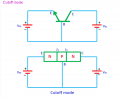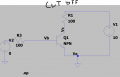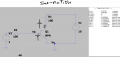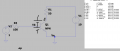#### MrChips

Joined Oct 2, 2009
28,551
The collector to emitter voltage is different.

#### yef smith

Joined Aug 2, 2020
498
Hello MrChips, i cant understand the logic of why in saturation state we have the maximal current. we have NP connected to PN. So we push the electron from emmiter buy putting lower potential to it and high potential to base. then the electrons reach the base and we want to pull them to the emitter ,so we put lower potential on the base and high potential to the collector. This is how they reach the collector. But this logic is for the active state. why in saturattion we get the maximal current? as i see it in active state we get maximal current because both diodes are set in such way that they pull electrons from emmiter to collector
Where is my ogic wrong? in active state we are doing our best to transfer electrons from emitter to base.

#### Audioguru again

Joined Oct 21, 2019
5,829
We do not talk about pushing and pulling electrons in a transistor. Instead we look at the datasheet and see the specs and graphs of the transistor's current gain (hFE) which is the amount of base current that causes the collector current. The ratio of the two currents is also affected by the base-emitter voltage, the collector-emitter voltage and the temperature.

When the base voltage is higher it causes the base-emitter current to be higher. The hFE of the transistor causes collector current which causes its voltage to decrease requiring a higher base-emitter voltage and current for the collector current to increase more. The ratio of the base current and collector current is not linear since near saturation the ratio of the two currents is much less than when active and not saturated.

The figure 1 graph in your transistor's datasheet shows that the hFE is high when the collector current is 50mA but the hFE is lower when the collector current is 100mA.
The figure 2 graph of saturation voltage shows an hFE of only 10 for good saturation instead of an hFE of hundreds when not saturated.

#### LvW

Joined Jun 13, 2013
1,649
....... But this logic is for the active state. why in saturattion we get the maximal current? as i see it in active state we get maximal current because both diodes are set in such way that they pull electrons from emmiter to collector
Where is my ogic wrong? in active state we are doing our best to transfer electrons from emitter to base.
* Saturation
: This operational mode is DEFINED for the case that the collector potential is LOWER than the base potential (due to a large voltage drop across the collector resistor). As a consequence, BOTH pn junctions (B_E and B-C) are forward biased and the base current IB (into the base node) is much larger than anticipated by the relation IB=IC/B which holds for quasi-linear (amplifying) operation only (see next point).

* (Quasi-)linear operation (active region): The collector current as well as the base current are both related to and controlled by the base-emitter voltage VBE. Of course, there is a (nearly) fixed relation between IB and IC (IB=IC/B), but this relation does not tell us anything about cause and effect - the current IC is NOT caused or controlled by IB.
It is the voltage VBE which determines both currents according to the well known exponential formula (Shockleys equation).

* DC bias: Due to the steep IC=f(VBE) characteristic a small change in BE will cause a pretty large change in Ic.
More than that, due to tolerances and temperature sensitivities of the BJT parameters it is common practice to use such gain stages with DC negative feedback only (in most cases: Emitter resistor RE). Such a DC stabilization method works best if we design a low-resistive base voltage divider.
In this case, the voltage divider provides a - more or less - "stiff" DC bias at the base node (and uncertainties of IB=IC/B play a minor role only).
Rule of thumb: Make the base current Ib at least (app.) 10 times smaller than the current through the divder).

Negative feedback always reduces the sensitivities of circuit properties to uncertainties in active parameters (applies also to opamps, of course). Therfore, in practice it is not very important if we assume VBE=0.65 volts or VBE=0.7 volts during calculations.

Last edited:

#### MrChips

Joined Oct 2, 2009
28,551
Hello MrChips, i cant understand the logic of why in saturation state we have the maximal current. we have NP connected to PN. So we push the electron from emmiter buy putting lower potential to it and high potential to base. then the electrons reach the base and we want to pull them to the emitter ,so we put lower potential on the base and high potential to the collector. This is how they reach the collector. But this logic is for the active state. why in saturattion we get the maximal current? as i see it in active state we get maximal current because both diodes are set in such way that they pull electrons from emmiter to collector
Where is my ogic wrong? in active state we are doing our best to transfer electrons from emitter to base.
You have it backwards. Saturation state does not produce maximum current.

If you drive the transistor ON hard enough the transistor cannot conduct more current. It is conducting the maximum current.
It transistor has reached a saturation state. "Saturation" is just a state that the transistor has reached.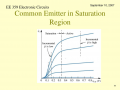#### WBahn

Joined Mar 31, 2012
28,514
Hello MrChips,i have switched to voltage divider configuration as shown bellow.
what is the strategy to calculate exactly the Vb number given by simulator?
To calculate the exact Vb number given by the simulator, you would need to pull out the parameters used by the transistor model and then use the mathematical model used by the simulator, with those parameters, to iteratively arrive at a solution in which the base voltage results in just the right amount of current being drawn out of the base-bias voltage divider to exactly match the base current at that same voltage. In general, this is a very complicated calculation.

The models used by simulators are much, much more complicated than the models we use for hand calculations -- though this does not automatically mean that they are more accurate since a poorly-characterized model is still poorly characterized, no matter how complex it is. But in either case the models are approximations to the real world and the results they produce are therefore only approximations to what you would actually see with the physical circuits.

For hand calculations we typically use one of five models. The first two are variations of the same one, namely that when the transistor is in the active region or in saturation that the Vbe is a fixed constant. In the simplest model we assume it is 0 V (a truly ideal transistor) and this is good enough for a quick analysis of a surprisingly large number of circuits. The second model just changes that ideal 0 V drop to a fixed Vbe. The that fixed value of Vbe depends on the type of transistor and perhaps on how it is being used. For most silicon small-signal transistors, a value of between 600 mV and 800 mV is pretty common, with perhaps 700 mV being the defacto "typical" value used. Most circuits are designed so that the exact value of Vbe has little impact on the behavior of the circuit, despite the fact that for circuits in the active region, it's the small variations in this voltage that allow the circuit to function. This is probably the model that is used more than any other for hand calculations. The next step up is a piecewise linear model in which we assume that the Vbe value is a specific value at a specific current (say 700 mV at a base current of 1 mA) and that it goes up or down linearly from there. This model is equivalent to the Vbe junction being a constant voltage source in series with a resistor. The step after that is to assume that the voltage change in Vbe is logarithmic with the current, typically that the voltage changed by about 60 mV for each factor of ten change in the base current. The final model, which is seldom used by hand, is the exponential Ebers-Moll model (which itself comes in several flavors of increasing complexity).

#### Audioguru again

Joined Oct 21, 2019
5,829
In post #19:
Since the collector resistor fed from 10V is 100 ohms and has 40mA in it then the resistor has 40mA x 100 ohms= 4V across it, then the collector voltage is 10V - 4V= 6V. The transistor is not saturated.

When its supply voltage is 10V and its collector resistor is 100 ohms, a saturated BC546B transistor has a "typical" collector voltage of 0.2V when its collector current is 100mA and its base current is 5mA. The base voltage and current in your schematic are very low. Some real transistors will not turn on, but the simulation guesses that a "typical" one will turn on.

You should not rely on the "typical" transistors in a simulation unless you buy thousands of that transistor, part number, test them all and select only the ones that have "typical" specs.